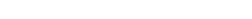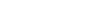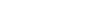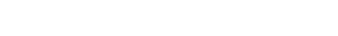How Cheenta works to ensure student success?
Explore the Back-Story

# Test of Mathematics Solution Subjective 82 - Inequality on four positive real numbersThis is a Test of Mathematics Solution Subjective 82 (from ISI Entrance). The book, Test of Mathematics at 10+2 Level is Published by East West Press. This problem book is indispensable for the preparation of I.S.I. B.Stat and B.Math Entrance.

Also visit: I.S.I. & C.M.I. Entrance Course of Cheenta

## Problem

Let a, b, c, d be positive real numbers such that abcd = 1. Show that## Solution

Concept: Inequality (see this link for some background information).

Using A.M. - G.M. inequality we see thatHenceThereforeThis is a Test of Mathematics Solution Subjective 82 (from ISI Entrance). The book, Test of Mathematics at 10+2 Level is Published by East West Press. This problem book is indispensable for the preparation of I.S.I. B.Stat and B.Math Entrance.

Also visit: I.S.I. & C.M.I. Entrance Course of Cheenta

## Problem

Let a, b, c, d be positive real numbers such that abcd = 1. Show that## Solution

Concept: Inequality (see this link for some background information).

Using A.M. - G.M. inequality we see thatHenceTherefore### Knowledge Partner